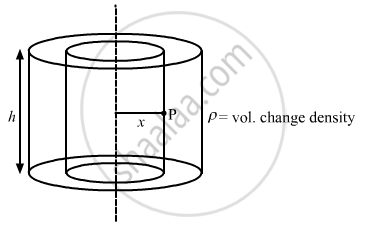Department of Pre-University Education, Karnataka course PUC Karnataka Science Class 12
Share

# A Long Cylindrical Volume Contains a Uniformly Distributed Charge of Density ρ. Find the Electric Field at a Point P Inside the Cylindrical Volume at a Distance X from Its Axis (See the Figure). - Physics

ConceptElectric Charges

#### Question

A long cylindrical volume contains a uniformly distributed charge of density ρ. Find the electric field at a point P inside the cylindrical volume at a distance x from its axis (see the figure).#### Solution

Given:-

Volume charge density inside the cylinder = ρ

Let the radius of the cylinder be r.

Let charge enclosed by the given cylinder be Q

Consider a Gaussian cylindrical surface of radius x and height h.

Let charge enclosed by the cylinder of radius x be q'.The charge on this imaginary cylinder can be found by taking the product of the volume charge density of the cylinder and the volume of the imaginary cylinder. Thus, q' = ρ( π x2 h)

From Gauss's Law,

oint  "E" . "ds" = ("q"_"en")/∈_0

"E" . 2 pi "xh" = (ρ( pi "x"^2 "h"))/ ∈_0

"E"  = (ρ"x")/(2∈_0)

Is there an error in this question or solution?

#### Video TutorialsVIEW ALL 

Solution A Long Cylindrical Volume Contains a Uniformly Distributed Charge of Density ρ. Find the Electric Field at a Point P Inside the Cylindrical Volume at a Distance X from Its Axis (See the Figure). Concept: Electric Charges.
S